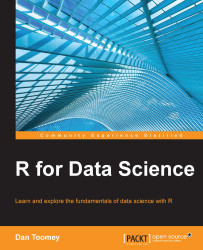•#### R for Data Science#### Overview of this book

R for Data ScienceCreditswww.PacktPub.comPrefaceFree Chapter
Data Mining PatternsData Mining SequencesText MiningData Analysis – Regression AnalysisData Analysis – CorrelationData Analysis – ClusteringData Visualization – R GraphicsData Visualization – PlottingData Visualization – 3DMachine Learning in ActionPredicting Events with Machine LearningSupervised and Unsupervised LearningIndex## Summary

In this chapter, we investigated machine learning in action using R. We learned about breaking up our dataset into a training and testing section. The examples showed how to use the `predict` method from our models. We generated models using linear regression, stepwise regression, k-nearest neighbor, Naïve Bayes, k-clustering, decision trees, neural net, and the random forest algorithms. We applied tests of the models' effectiveness.

In the next chapter, we will cover predicting events with machine learning.